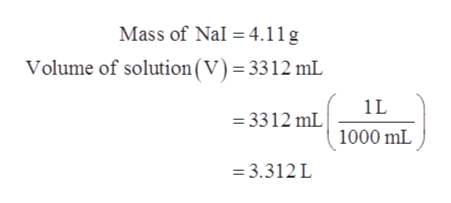# Calculate the molarity of a solution prepared by dissolving 4.11g of NaI in enough water to prepare 3312 mL od solution.

Question
6 views

Calculate the molarity of a solution prepared by dissolving 4.11g of NaI in enough water to prepare 3312 mL od solution.

check_circle

Step 1

Molarity is the described as the number of moles present in 1 L solution. Molarity is expressed in mol/L. The mathematical expression is shown below.

Step 2

Given datahelp_outlineImage TranscriptioncloseMass of Nal = 4.11g Volume of solution(V) = 3312 mL 1L = 3312 mL 1000 mL = 3.312L fullscreen
Step 3

The molar mass of NaI is 149.89 g/mol.

The number of ...

### Want to see the full answer?

See Solution

#### Want to see this answer and more?

Solutions are written by subject experts who are available 24/7. Questions are typically answered within 1 hour.*

See Solution
*Response times may vary by subject and question.
Tagged in

### Physical Chemistry